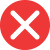22nd

Correct spelling, explanation: as -nd is the suffix for the ordinal form of number 2, every number that ends with two will have this suffix (as in the ordinal number full form: second). -th is a usual suffix for ordinal numbers, but not all of them. Therefore 22nd is correct and 22th is wrong.

Definition of 22nd:
numeral, the ordinal number between 21st and 23rd
Mary got a car for her 22nd birthday.
I was 22nd in line for the doctor today so I was waiting a long time.22th

Incorrect spelling, explanation: in this case, we don’t use the suffix -th (which is usually used to create ordinal numbers), because the number 22 ends with two, which means its ordinal form will end with second, therefore with the suffix -nd in its numeral form. This is why 22th is an incorrect form. The right one is 22nd.22st

Incorrect spelling22rd

Incorrect spelling